Thread: Eric Dollard View Single Postt-rexSenior Member Join Date: Aug 2011 Location: In the bushes Posts: 422
The Law of E.M. Induction, Part 6 (2 of 3)

(4) The total magnetic induction can be sub-divided into a pair of independent factors.

One, the intensity of the magneto-motive motive force, this maintaining the quantity of magnetism, Force, i, in ampere.

Two, the concentration , or inductance, containing the quantity of magnetism, Concentration, L, in henry.

These two factors are related thru the Law of Magnetic Proportion, given by,Where, i, is the ampere of current. Transposing gives the relation for magnetic induction as,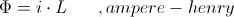Hence the total magnetic induction is the product of two Independant Parameters, each of which contributes to the induction. Each of these two parameters can be varied separately. The E.M.F. is developed thru the resulting variation of the total magnetic induction.

The total energy contained in the magnetic induction is given by the relation,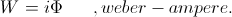It was given that,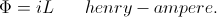Substituting this into weber-ampere gives,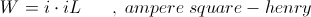And this leads to the expression of magnetic energy as,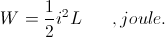(5) The quantity of magnetic induction is made to vary by variation of the current or the variation of inductance, or by the variation of both. This variation of magnetism that results from the parameter variation gives rise to a variation of the quantity of stored energy contained in the magnetic induction. The E.M.F. developed by the variation of magnetism is the means by which the stored energy can either leave or enter its magnetic form. It is then the current, or M.M.F., represents the potential energy stored in the magnetic field, and the E.M.F. represents the kinetic energy given or taken by the magnetic field.

This is analogous to the dielectric field. Here it is the electro-static potential, e, in volt that represents the potential energy of the field. The displacement current, I, in ampere then represents the kinetic energy taken or given to the dielectric field. Hence,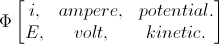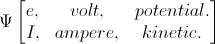Therefore where it is that the voltage, e, is the electro-static potential, it is the M.M.F. (and its current, i,) is the magneto-static potential.

Both potentials in themselves do not represent energy, only the force required to maintain this energy in a static, or potential, state.

(6) The M.M.F. is the force holding the magnetism in place. A stationary magnetic field needs a continuous current to remain stationary.

The inductance is the holder of the magnetism. An invariant inductance can hold a stationary magnetic field of induction in place.

Variation of either the M.M.F. or the inductance requires the magnetism move. This gives rise to an E.M.F. of energy transfer, this as a result of a time rate of change in the quantity of magnetism. The E.M.F. is directly proportional to this change, the quicker the change, the larger the E.M.F.

Neither the M.M.F., nor the inductance, represent energy. They are only factors of the induction, parts of a whole. While each parameter effects the magnetism as a whole, the effects of each are not interchangeable, and this needs to be taken into account regarding the Law of Energy Continuity.

(7) In the static reactance coil it is the intensity of force, as a current, that is made to vary. This variation is expressed in ampere per second,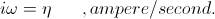Where,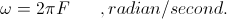The inductance of the reactance coil is constant, or invariant in this situation. Hence the E.M.F. developed by the reactance coil is derived from the time rate of current variation only. This is expressed by the relation,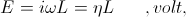Where,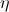, ampere-second. It is customary however to express this relation using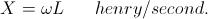and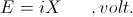But here in the reactance coil the inductance is in-variant, no such henry per second exists. It is the ampere per second which acts here. Hence in its common expression the term reactance can be misleading.
__________________
SUPPORT ERIC DOLLARD'S WORK AT EPD LABORATORIES, INC.

Purchase Eric Dollard's Books & Videos - 70% of the sale goes to Eric and EPD Laboratories: Eric Dollard Books & Videos
Donate by Paypal: Donate to EPD Laboratories

Last edited by t-rex; 07-25-2012 at 03:00 AM.